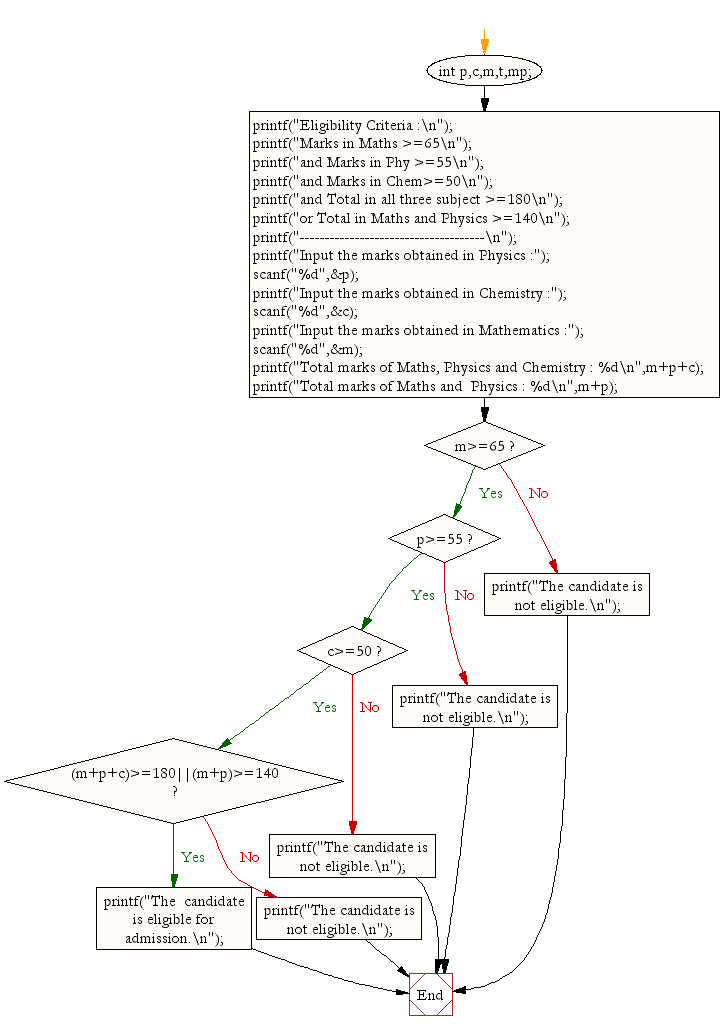﻿ C : Find eligibility for admission using Nested If Statement

# C Exercises: Find eligibility for admission using Nested If Statement

## C Conditional Statement: Exercise-10 with Solution

Write a C program to determine eligibility for admission to a professional course based on the following criteria:
Marks in Maths >=65
Marks in Phy >=55
Marks in Chem>=50
Total in all three subject >=190
or
Total in Math and Physics >=140

Sample Solution:

C Code:

``````#include <stdio.h>
void main()
{  int p,c,m,t,mp;

printf("Eligibility Criteria :\n");
printf("Marks in Maths >=65\n");
printf("and Marks in Phy >=55\n");
printf("and Marks in Chem>=50\n");
printf("and Total in all three subject >=190\n");
printf("or Total in Maths and Physics >=140\n");
printf("-------------------------------------\n");

printf("Input the marks obtained in Physics :");
scanf("%d",&p);
printf("Input the marks obtained in Chemistry :");
scanf("%d",&c);
printf("Input the marks obtained in Mathematics :");
scanf("%d",&m);
printf("Total marks of Maths, Physics and Chemistry : %d\n",m+p+c);
printf("Total marks of Maths and  Physics : %d\n",m+p);

if (m>=65)
if(p>=55)
if(c>=50)
if((m+p+c)>=190||(m+p)>=140)
printf("The  candidate is eligible for admission.\n");
else
printf("The candidate is not eligible.\n");
else
printf("The candidate is not eligible.\n");
else
printf("The candidate is not eligible.\n");
else
printf("The candidate is not eligible.\n");
}
```
```

Sample Output:

```Eligibility Criteria :
Marks in Maths >=65
and Marks in Phy >=55
and Marks in Chem>=50
and Total in all three subject >=190
or Total in Maths and Physics >=140
-------------------------------------
Input the marks obtained in Physics :65
Input the marks obtained in Chemistry :51
Input the marks obtained in Mathematics :72
Total marks of Maths, Physics and Chemistry : 188
Total marks of Maths and  Physics : 137
The candidate is not eligible.
```

Flowchart:C Programming Code Editor:

Improve this sample solution and post your code through Disqus.

What is the difficulty level of this exercise?

Test your Programming skills with w3resource's quiz.

﻿

## C Programming: Tips of the Day

Returning an array using C

You can't return arrays from functions in C. You also can't (shouldn't) do this:

```char *returnArray(chararray []){
char returned ;
//methods to pull values from array, interpret them, and then create new array
return&(returned); //is this correct?
}
```

returned is created with automatic storage duration and references to it will become invalid once it leaves its declaring scope, i.e., when the function returns.

You will need to dynamically allocate the memory inside of the function or fill a preallocated buffer provided by the caller.

Option 1:

dynamically allocate the memory inside of the function (caller responsible for deallocating ret)

```char *foo(int count){
char *ret = malloc(count);
if(!ret)
returnNULL;

for(int i = 0; i < count; ++i)
ret[i] = i;

return ret;
}
```

Call it like so:

```intmain(){
char *p = foo(10);
if(p) {
// do stuff with p
free(p);
}

return0;
}
```

Option 2:

fill a preallocated buffer provided by the caller (caller allocates buf and passes to the function)

```voidfoo(char *buf, int count){
for(int i = 0; i < count; ++i)
buf[i] = i;
}
```

And call it like so:

```intmain(){
char arr = {0};
foo(arr, 10);
// No need to deallocate because we allocated
// arr with automatic storage duration.
// If we had dynamically allocated it
// (i.e. malloc or some variant) then we
// would need to call free(arr)
}
```

Ref :https://bit.ly/3hKUill

We are closing our Disqus commenting system for some maintenanace issues. You may write to us at reach[at]yahoo[dot]com or visit us at Facebook